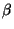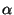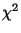IdentifiantMot de passe
Mot de passe oublié ?
20.2 Special random number distributions

# 20.2 Special random number distributions

## 20.2.1 Random floating point number distributions

 beta( a, b, shape=[])
The beta function returns an array of the specified shape that contains Float numbers-distributed with-parameter a and-parameter b.

The a and b arguments can be arrays. If this is the case, and the output shape is specified, a and b are broadcasted if their dimensions are not equal to shape. If shape is not specified, the shape of the output is equal to the shape of a and b after broadcasting.

If no shape is specified, and a and b are scalars, a single value is returned.

 chi_square( df, shape=[])
The chi_square function returns an array of the specified shape that contains Float numbers with the-distribution with df degrees of freedom.

The df argument can be an array. If this is the case, and the output shape is specified, df is broadcasted if its dimensions are not equal to shape. If shape is not specified, the shape of the output is equal to the shape of df.

If no shape is specified, and df is a scalar, a single value is returned.

 exponential( mean, shape=[])
The exponential function returns an array of the specified shape that contains Float numbers exponentially distributed with the specified mean.

The mean argument can be an array. If this is the case, and the output shape is specified, mean is broadcasted if its dimensions are not equal to shape. If shape is not specified, the shape of the output is equal to the shape of mean.

If no shape is specified, and mean is a scalar, a single value is returned.

 F( dfn, dfd, shape=[])
The F function returns an array of the specified shape that contains Float numbers with the F-distribution with dfn degrees of freedom in the numerator and dfd degrees of freedom in the denominator.

The dfn and dfd arguments can be arrays. If this is the case, and the output shape is specified, dfn and dfd are broadcasted if their dimensions are not equal to shape. If shape is not specified, the shape of the output is equal to the shape of dfn and dfd after broadcasting.

If no shape is specified, and dfn and dfd are scalars, a single value is returned.

 gamma( a, r, shape=[])
The gamma function returns an array of the specified shape that contains Float numbers-distributed with location parameter a and distribution shape parameter r.

The a and r arguments can be arrays. If this is the case, and the output shape is specified, a and r are broadcasted if their dimensions are not equal to shape. If shape is not specified, the shape of the output is equal to the shape of a and r after broadcasting.

If no shape is specified, and a and r are scalars, a single value is returned.

 multivariate_normal( mean, cov, shape=[])
The multivariate_normal function takes a one dimensional array argument mean and a two dimensional array argument cov. Suppose the shape of mean is (n,). Then the shape of cov must be (n,n). The function returns an array of Floats.

The effect of the shape parameter is:

• If no shape is specified, then an array with shape (n,) is returned containing a vector of numbers with a multivariate normal distribution with the specified mean and covariance.
• If shape is specified, then an array of such vectors is returned. The shape of the output is shape.append((n,)). The leading indices into the output array select a multivariate normal from the array. The final index selects one number from within the multivariate normal.
In either case, the behavior of multivariate_normal is undefined if cov is not symmetric and positive definite.

 normal( mean, std, shape=[])
The normal function returns an array of the specified shape that contains Float numbers normally distributed with the specified mean and standard deviation std.

The mean and std arguments can be arrays. If this is the case, and the output shape is specified, mean and std are broadcasted if their dimensions are not equal to shape. If shape is not specified, the shape of the output is equal to the shape of mean and std after broadcasting.

If no shape is specified, and mean and std are scalars, a single value is returned.

 noncentral_chi_square( df, nonc, shape=[])
The noncentral_chi_square function returns an array of the specified shape that contains Float numbers with the-distribution with df degrees of freedom and noncentrality parameter nconc.

The df and nonc arguments can be arrays. If this is the case, and the output shape is specified, df and nonc are broadcasted if their dimensions are not equal to shape. If shape is not specified, the shape of the output is equal to the shape of df and nonc after broadcasting.

If no shape is specified, and df and nonc are scalars, a single value is returned.

 noncentral_F( dfn, dfd, nconc, shape=[])
The noncentral_F function returns an array of the specified shape that contains Float numbers with the F-distribution with dfn degrees of freedom in the numerator, dfd degrees of freedom in the denominator, and noncentrality parameter nconc.

The dfn, dfd and nonc arguments can be arrays. If this is the case, and the output shape is specified, dfn, dfd and nonc are broadcasted if their dimensions are not equal to shape. If shape is not specified, the shape of the output is equal to the shape of dfn, dfd and nonc after broadcasting.

If no shape is specified, and dfn, dfd and nonc are scalars, a single value is returned.

 standard_normal( shape=[])
The standard_normal function returns an array of the specified shape that contains Float numbers normally (Gaussian) distributed with mean zero and variance and standard deviation one.

If no shape is specified, a single number is returned.

 F( dfn, dfd, shape=[])
Returns array of F distributed random numbers with dfn degrees of freedom in the numerator and dfd degrees of freedom in the denominator.

 noncentral_F( dfn, dfd, nconc, shape=[])
Returns array of noncentral F distributed random numbers with dfn degrees of freedom in the numerator and dfd degrees of freedom in the denominator, and noncentrality parameter nconc.

## 20.2.2 Random integer number distributions

 binomial( trials, p, shape=[])
The binomial function returns an array with the specified shape that contains Integer numbers with the binomial distribution with trials and event probability p. In other words, each value in the returned array is the number of times an event with probability p occurred within trials repeated trials.

The trials and p arguments can be arrays. If this is the case, and the output shape is specified, trials and p are broadcasted if their dimensions are not equal to shape. If shape is not specified, the shape of the output is equal to the shape of trials and p after broadcasting.

If no shape is specified, and trials and p are scalars, a single value is returned.

 negative_binomial( trials, p, shape=[])
The negative_binomial function returns an array with the specified shape that contains Integer numbers with the negative binomial distribution with trials and event probability p.

The trials and p arguments can be arrays. If this is the case, and the output shape is specified, trials and p are broadcasted if their dimensions are not equal to shape. If shape is not specified, the shape of the output is equal to the shape of trials and p after broadcasting.

If no shape is specified, and trials and p are scalars, a single value is returned.

 multinomial( trials, probs, shape=[])
The multinomial function returns an array with that contains integer numbers with the multinomial distribution with trials and event probabilities given in probs. probs must be a one dimensional array. There are len(probs)+1 events. probs[i] is the probability of the i-th event for 0<=i<len(probs). The probability of event len(probs) is 1.-Numeric.sum(prob).

The function returns an integer array of shape shape + (len(probs)+1,). If shape is not specified this is one multinomially distributed vector of shape (len(prob)+1,). Otherwise each returnarray[i,j,...,:] is an integer array of shape (len(prob)+1,) containing one multinomially distributed vector.

 poisson( mean, shape=[])
The poisson function returns an array with the specified shape that contains Integer numbers with the Poisson distribution with the specified mean.

The mean argument can be an array. If this is the case, and the output shape is specified, mean is broadcasted if its dimensions are not equal to shape. If shape is not specified, the shape of the output is equal to the shape of mean.

If no shape is specified, and mean is a scalar, a single value is returned.

Send comments to the NumArray community.